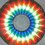# Some more proof problems (from CMI)....

1) If $f(x)=\frac{x^n}{n!}+\frac{x^(n-1)}{(n-1)!}...x+1$ then prove that f cannot have repeated roots.

This one I did not expect it:

2) If f(x) is a polynomial with integer coefficients such that f(0)=1 and f(1)=5, then prove that f cannot have integer roots.

Check out my set hereNote by Vishnu C
6 years, 2 months ago

This discussion board is a place to discuss our Daily Challenges and the math and science related to those challenges. Explanations are more than just a solution — they should explain the steps and thinking strategies that you used to obtain the solution. Comments should further the discussion of math and science.

When posting on Brilliant:

• Use the emojis to react to an explanation, whether you're congratulating a job well done , or just really confused .
• Ask specific questions about the challenge or the steps in somebody's explanation. Well-posed questions can add a lot to the discussion, but posting "I don't understand!" doesn't help anyone.
• Try to contribute something new to the discussion, whether it is an extension, generalization or other idea related to the challenge.

MarkdownAppears as
*italics* or _italics_ italics
**bold** or __bold__ bold
- bulleted- list
• bulleted
• list
1. numbered2. list
1. numbered
2. list
Note: you must add a full line of space before and after lists for them to show up correctly
paragraph 1paragraph 2

paragraph 1

paragraph 2

[example link](https://brilliant.org)example link
> This is a quote
This is a quote
    # I indented these lines
# 4 spaces, and now they show
# up as a code block.

print "hello world"
# I indented these lines
# 4 spaces, and now they show
# up as a code block.

print "hello world"
MathAppears as
Remember to wrap math in $$ ... $$ or $ ... $ to ensure proper formatting.
2 \times 3 $2 \times 3$
2^{34} $2^{34}$
a_{i-1} $a_{i-1}$
\frac{2}{3} $\frac{2}{3}$
\sqrt{2} $\sqrt{2}$
\sum_{i=1}^3 $\sum_{i=1}^3$
\sin \theta $\sin \theta$
\boxed{123} $\boxed{123}$

Sort by:

For 1., you can use the fact that if $f(x)$ has a repeated root, then $f'(x)$ has the same root, say $a$ .

This implies that $f(a) - f'(a) = 0 \implies \dfrac{a^{n-1}}{(n-1)!} = 0 \implies a = 0$. But $0$ is obviously not a root of $f(x)$.Therefore, there exists no repeated root of $f(x)$.

For 2, you can see that modulo 2, $f(a) \equiv f(1) , f(0) \equiv 1$( depending on whether a is odd or even). for all integral $a$. But, if a integral root $b$ did exist, that would imply that $f(b) = 0 \equiv 0$, which leads to a contradiction

- 6 years, 2 months ago

Ya. I used the exact same methods for both the questions. Great minds think alike ; )

- 6 years, 2 months ago

1. We start by inducting on $n$. Let $f_n(x) = \sum_{k=0}^n \frac{x^k}{k!}$
Let's see the base case, where $n=1$ $f_1(x) = 1+x =0 \Rightarrow x=-1$ $f_1(x)$ has only $1$ root and thus does not have repeated roots.

Let us assume that $f_n(x) = 1+x+\frac{x^2}{2!}+\ldots+\frac{x^n}{n!}$ has no repeated roots.

We now study the function $f_{n+1}(x)$

$f_{n+1}(x) = 1+x+\frac{x^2}{2!}+\ldots+\frac{x^n}{n!}+\frac{x^{n+1}}{(n+1)!}$

Observe the derivative of $f_{n+1}(x)$ $f_{n+1}^{\prime}(x) = 1+x+\frac{x^2}{2!}+\ldots+\frac{x^n}{n!}$

Clearly, $f_{n+1}^{\prime}(x)$ has no repeated roots and must have $n$ distinct roots by our inductive hypothesis. Therefore, by Rolle's Theorem, we must have $n+1$ distinct roots of $f_{n+1}(x)$. And thus proved.

- 6 years, 2 months ago

- 6 years, 2 months ago

ok these ones are somewhat hard to understand I should say ...

- 6 years, 2 months ago

I've posted some more proof notes.

- 6 years, 2 months ago

The first one can be done with the help of LMV or Rolles i guess??

- 6 years, 2 months ago

The first one is pretty simple. I don't like using LMV or Rolle's theorem, or at least their terms in anything that can be solved with a rough sketch. Although I don't use it, my method is pretty much related to it. If the function has repeated roots, then it's derivative also has a root at that point. If that's the case, then the $x^n/n!$ factor in the function has no consequence in altering the value taken by the function. In that case, x must have a repeated root at 0. But substituting 0 in the place of x gives you 1. So 0 is not a root and the function doesn't have any repeated roots.

- 6 years, 2 months ago